# RS Aggarwal Solutions for Class 8 Maths Chapter 2 - Exponents Exercise 2B

In order to excel in the exams for Class 8, students can view and download the solutions of Exercise 2B available here. At BYJU’S, the subject experts solve the questions with the utmost care, giving proper knowledge to help solve the questions. It will help students to solve the exercise problems without any difficulties. This exercise provides in depth knowledge about the basic concepts of exponents as well as numbers in standard form.

By practising the RS Aggarwal Solutions for Class 8, students will be able to grasp the concepts perfectly.

## Download PDF of RS Aggarwal Solutions for Class 8 Maths Chapter 2 – Exponents Exercise 2B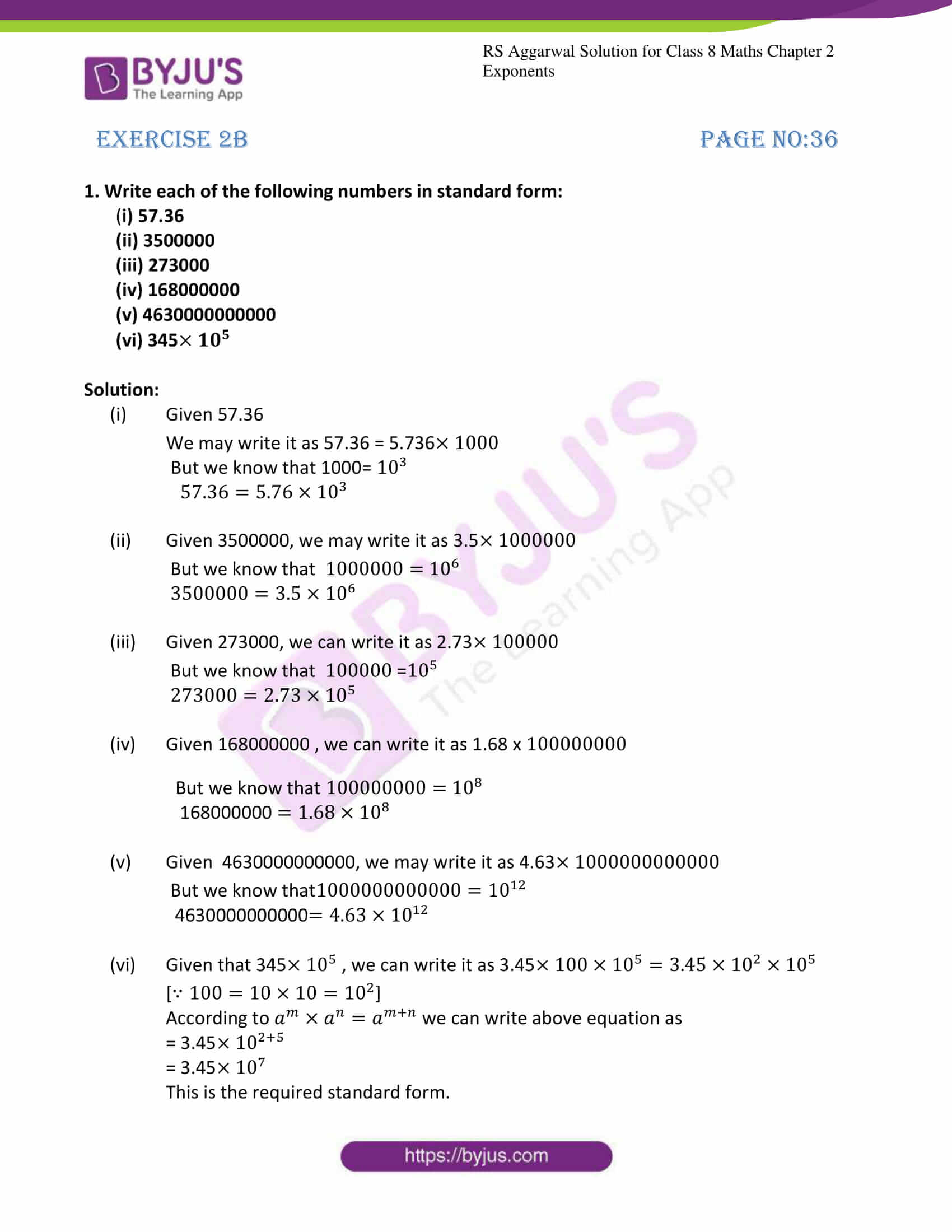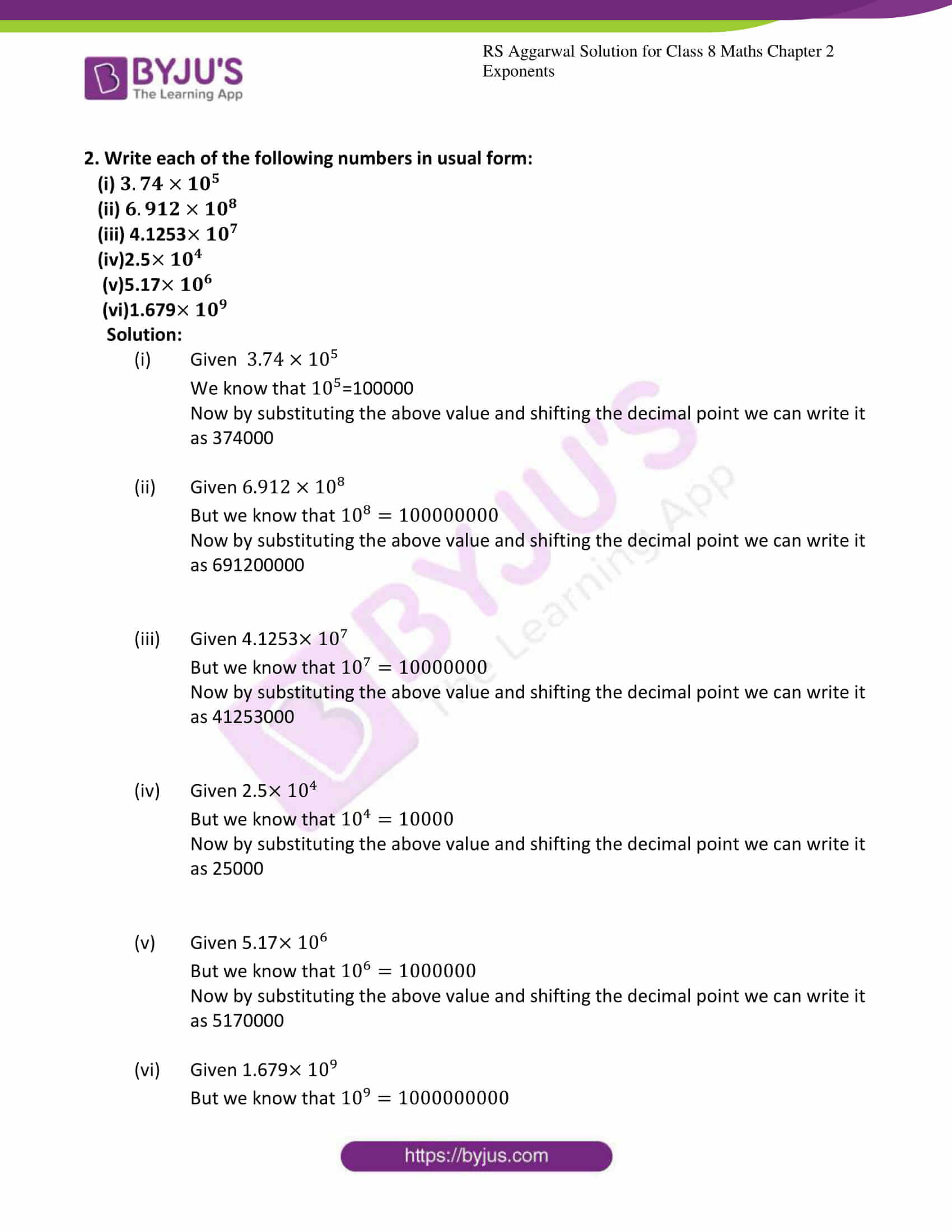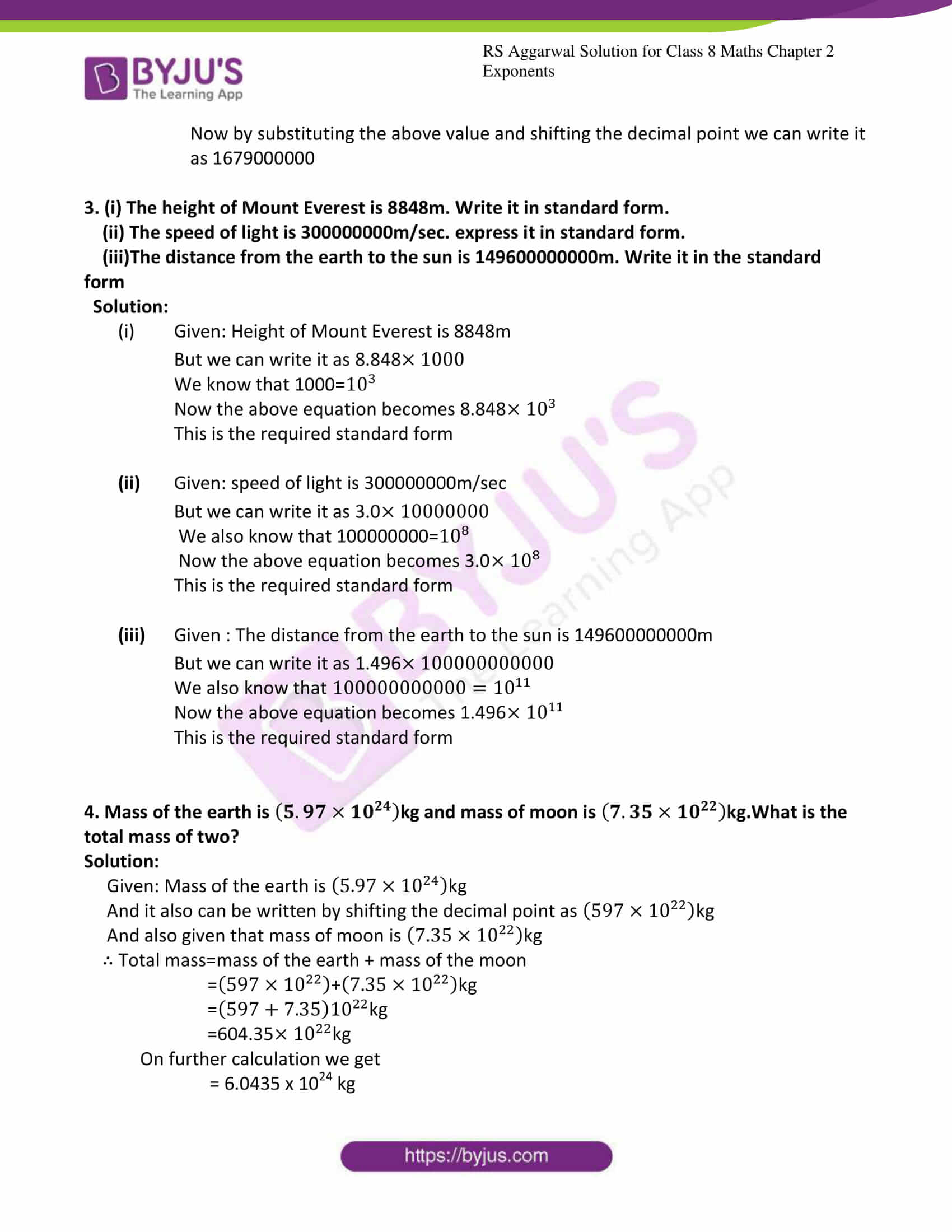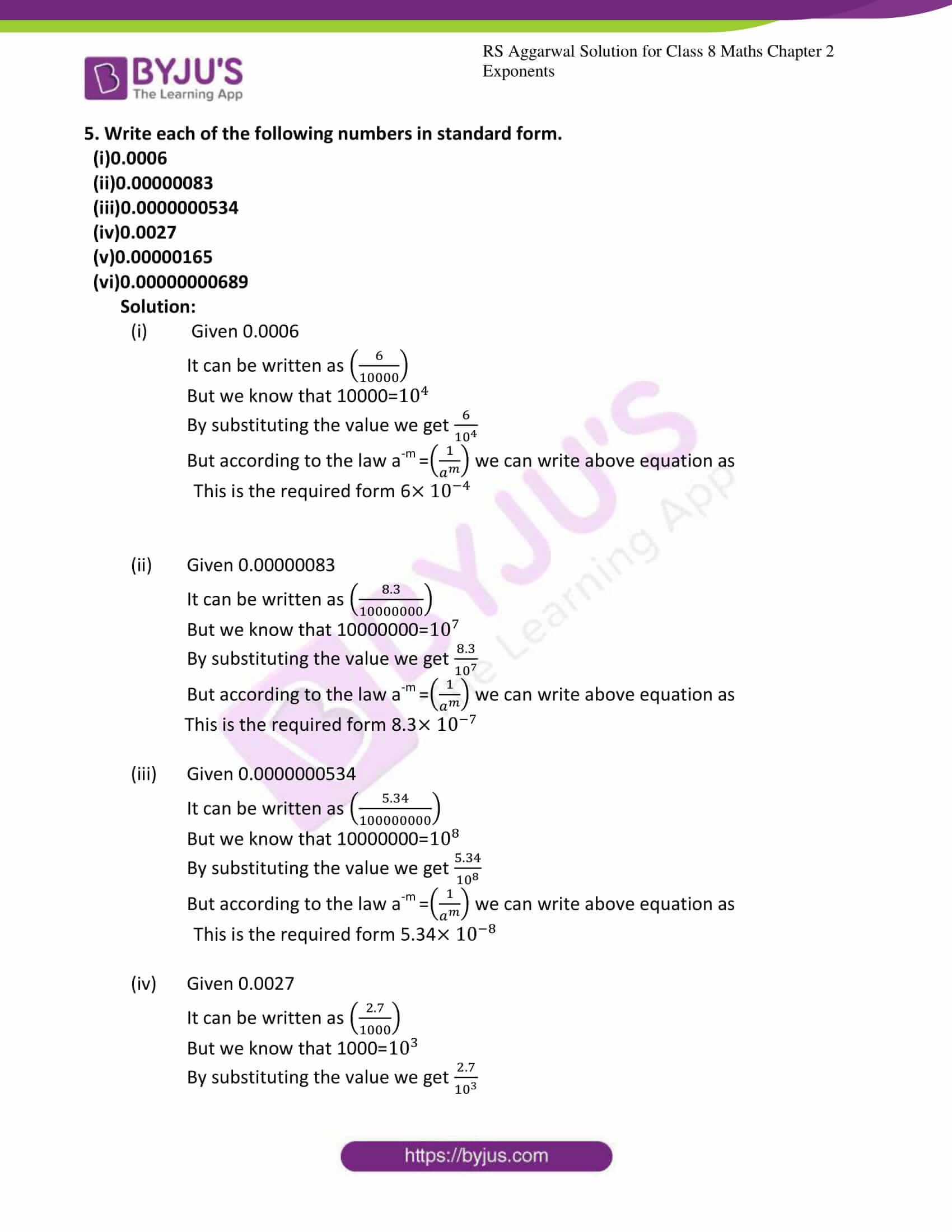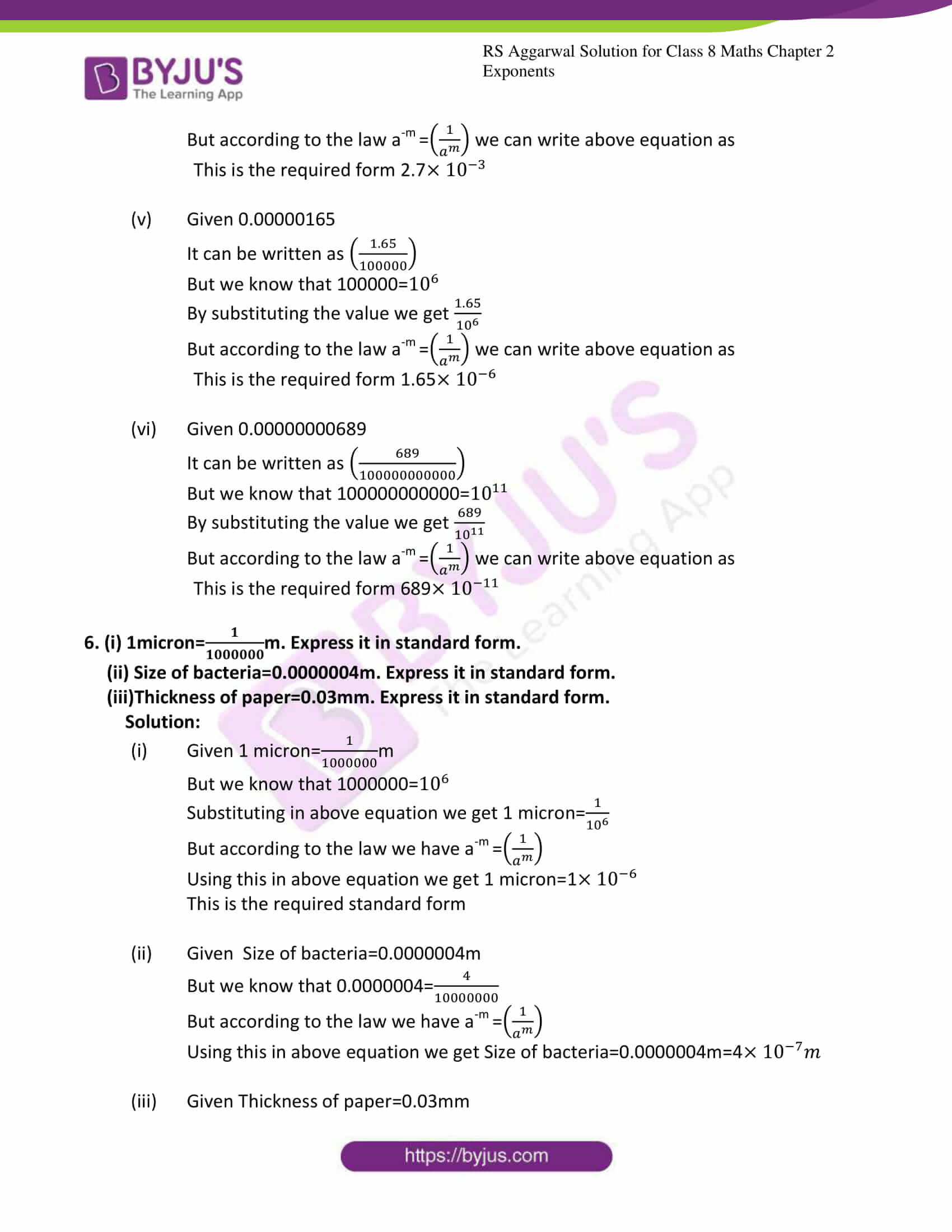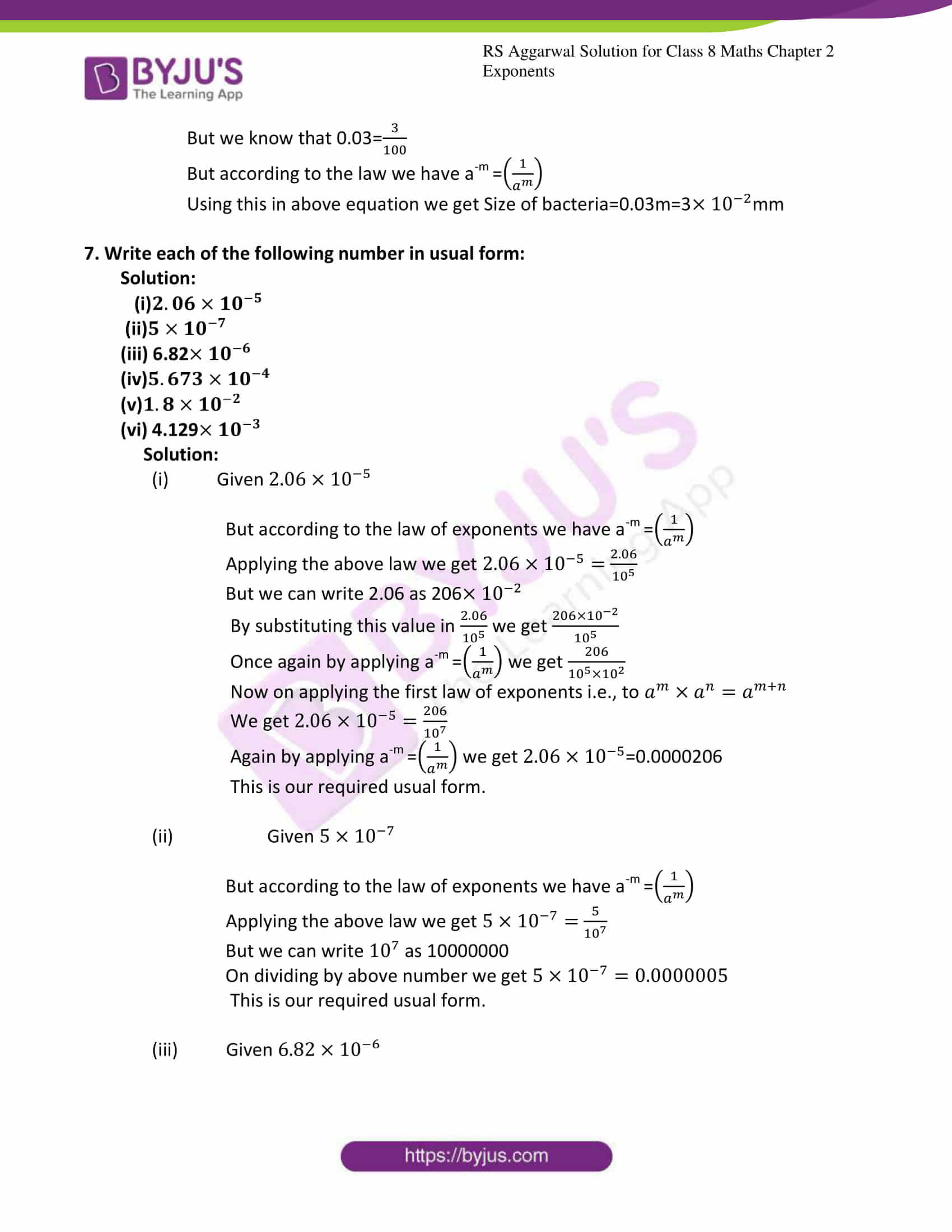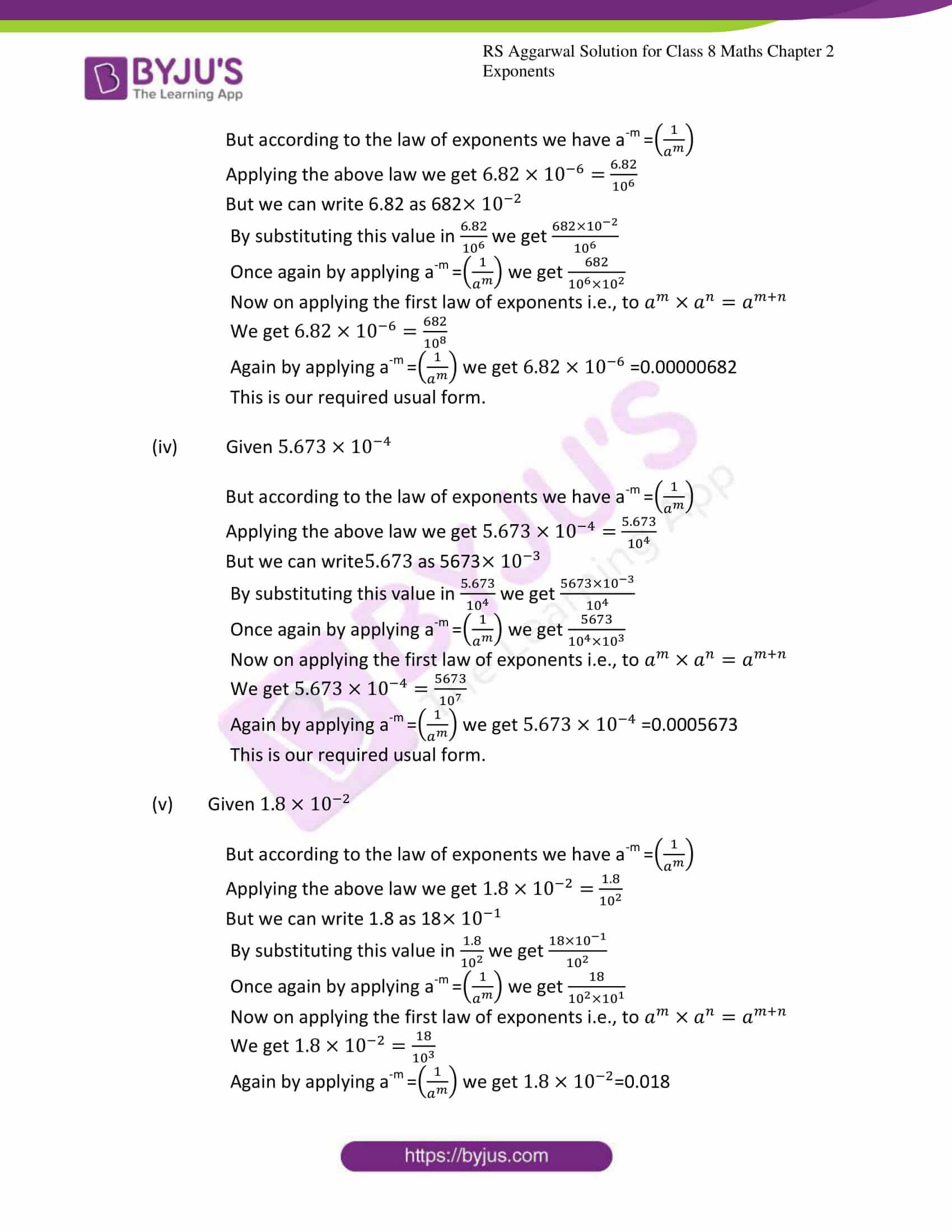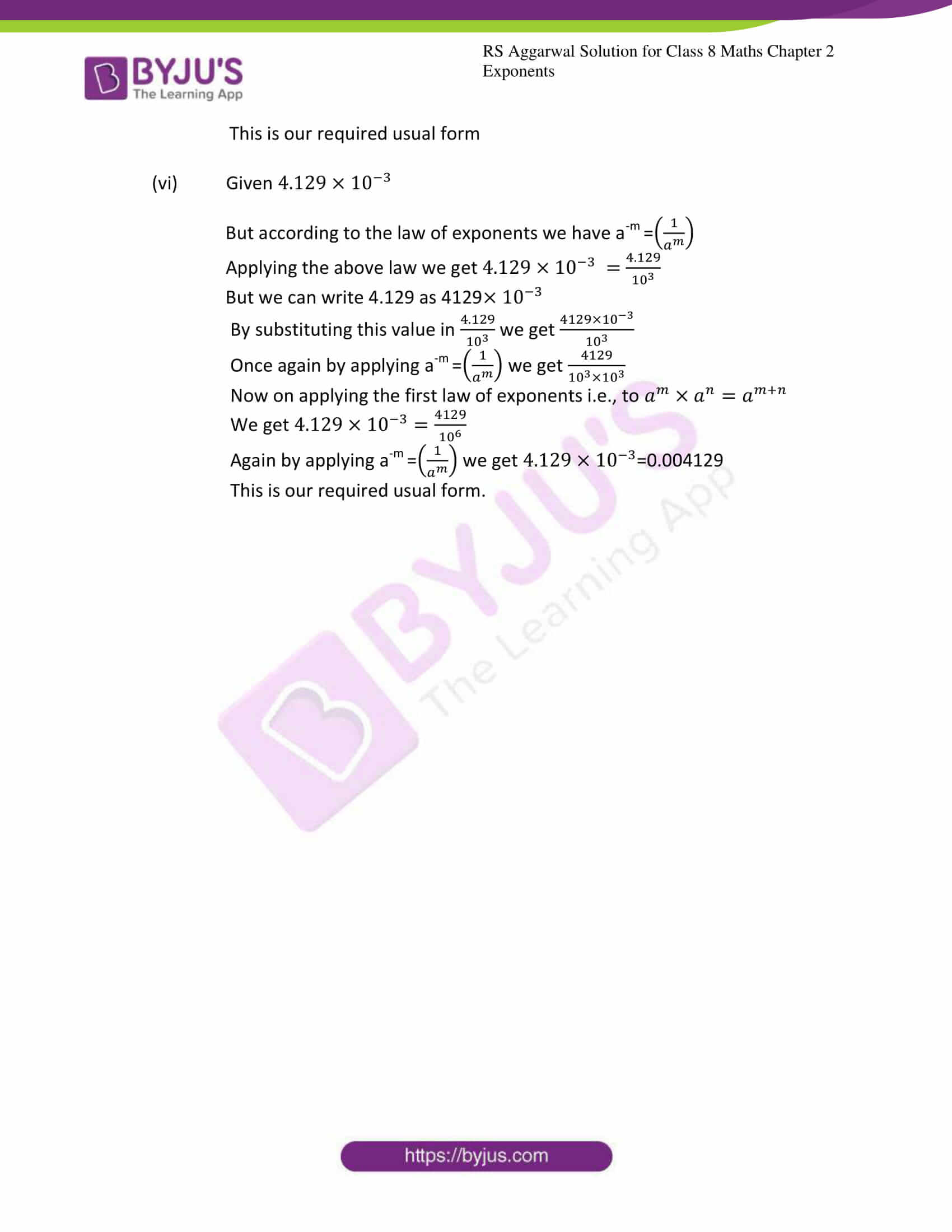### Access answers to Maths RS Aggarwal Solutions for Class 8 Chapter 2 – Exponents Exercise 2B

1. Write each of the following numbers in standard form:

(i) 57.36

(ii) 3500000

(iii) 273000

(iv) 168000000

(v) 4630000000000

(vi) 345 x 105

Solution: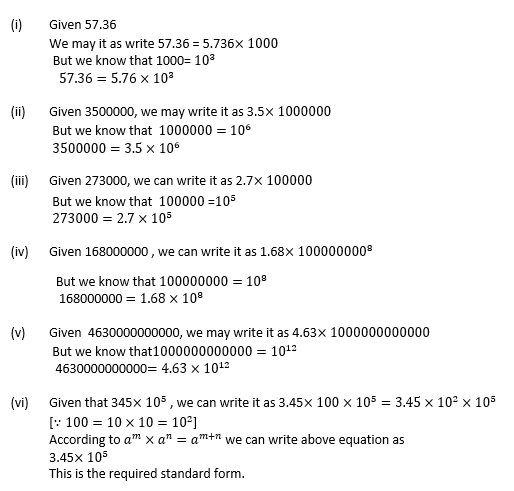2. Write each of the following numbers in usual form:

(i) 3.74 × 105

(ii) 6.912 × 108

(iii) 4.1253 × 107

(iv) 2.5 × 104

(v) 5.17 × 106

(vi) 1.679 × 109

Solution: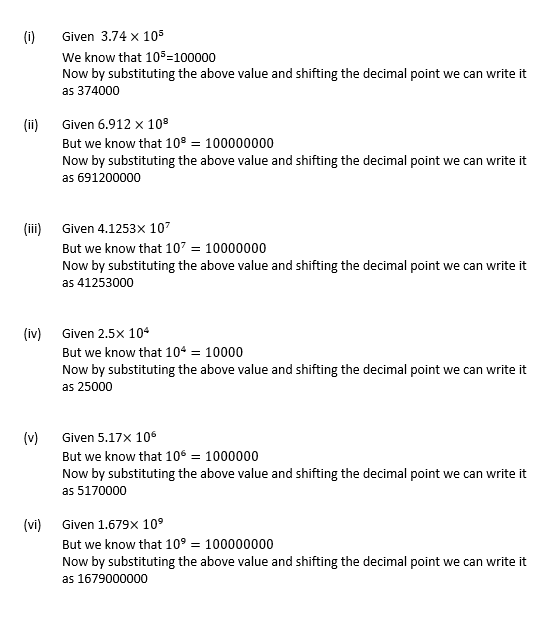3. (i) The height of Mount Everest is 8848m. Write it in standard form.

(ii) The speed of light is 300000000m/sec. express it in standard form.

(iii)The distance from the earth to the sun is 149600000000m. Write it in the standard form

Solution: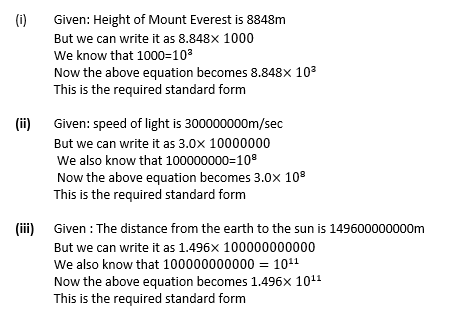4. Mass of the earth is (5.97 × 1024)kg and mass of moon is (7.35 × 1022) kg.What is the total mass of two?

Solution: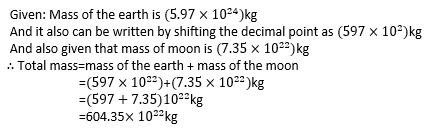5. Write each of the following numbers in standard form.

(i)0.0006

(ii)0.00000083

(iii)0.0000000534

(iv)0.0027

(v)0.00000165

(vi)0.00000000689

Solution: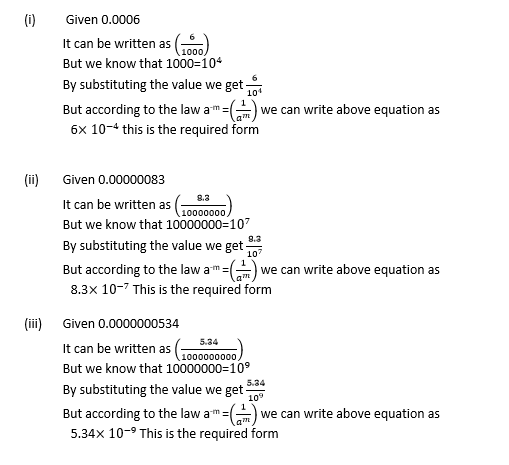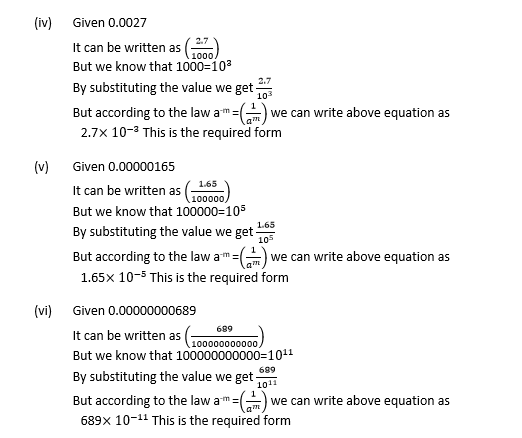6. (i) 1micron = 1/1000000 m. Express it in standard form.

(ii) Size of bacteria=0.0000004m. Express it in standard form.

(iii)Thickness of paper=0.03mm. Express it in standard form.

Solution: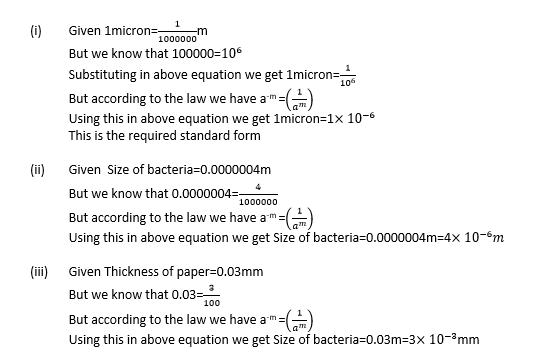7. Write each of the following number in usual form:

(i) 2.06 × 10-5

(ii) 5 × 10-7

(iii) 6.82 × 10-6

(iv) 5.673 × 10-4

(v) 1.8 × 10-2

(vi)4.129 × 10-3

Solution: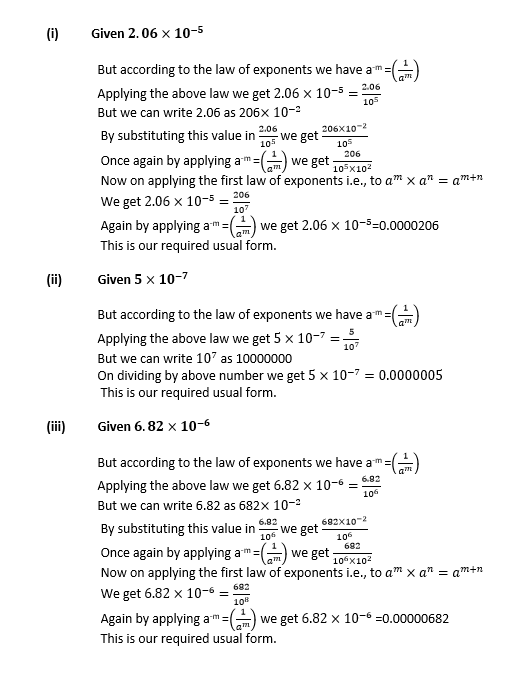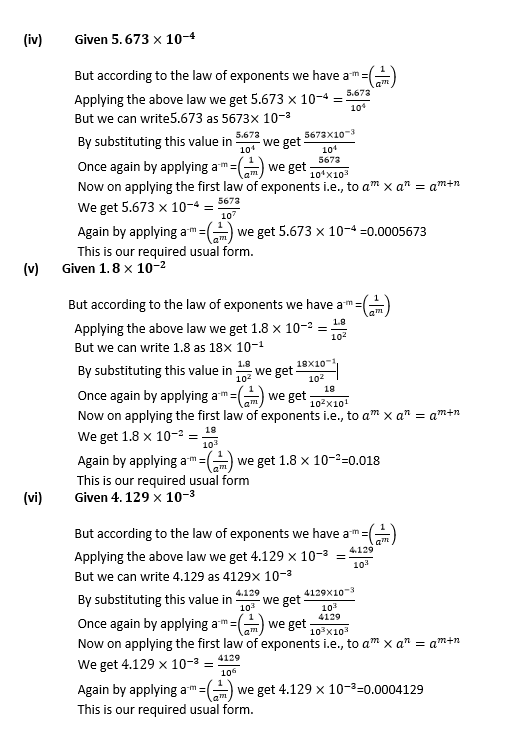### Access other exercises of RS Aggarwal Solutions for Class 8 Maths Chapter 2 – Exponents

Exercise 2A Solutions 13 questions

Exercise 2C Solutions 17 questions

## RS Aggarwal Solutions for Class 8 Maths Chapter 2 – Exponents Exercise 2B

Exercise 2B of RS Aggarwal Solutions for Chapter 2, Exponents, deals with the basic concepts related to exponents. This exercise mainly deals with the laws of exponents as per the latest syllabus of prescribed board. Some of the topics focused upon in exercise 2B include the following.

• Numbers in standard form
• Expressing very large numbers in standard form
• Expressing very small numbers in standard form

The RS Aggarwal Solutions can help the students in practising and learning each and every concept as it provides solutions to all questions asked in the RS Aggarwal textbook.

1. Anjali